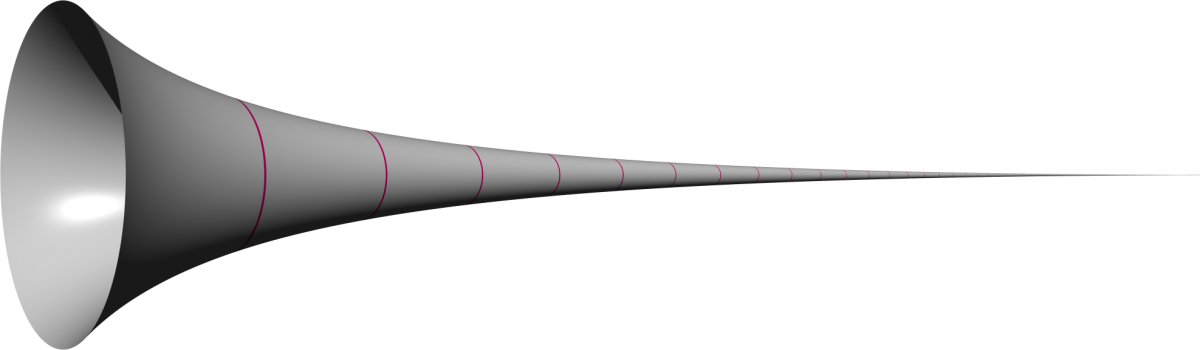# There’s an infinitely long “horn” that has a finite volume but an infinite surface areaMoving ahead to a problem posed in the 17th century, we’ve got one of many paradoxes related to infinity and geometry.

“Gabriel’s Horn” is formed by taking the curve y = 1/x and rotating it around the horizontal axis, as shown in the picture. Using techniques from calculus that make it possible to calculate areas and volumes of shapes constructed this way, it’s possible to see that the infinitely long horn actually has a finite volume equal to π, but an infinite surface area.

As stated in the MathWorld article on the horn, this means that the horn could hold a finite volume of paint but would require an infinite amount of paint to cover its entire surface.